# High School Math : Understanding Square Roots

## Example Questions

### Example Question #1 : Understanding Square Roots

Simplify the expression. Find the positive solution only.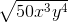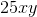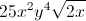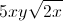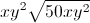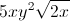Explanation:

When working in square roots, each component can be treated separately.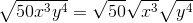Now, we can simplify each term.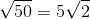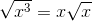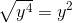Combine the simplified terms to find the answer. Anything outside of the square root is combined, while anything under the root is combined under the root.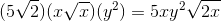### All High School Math Resources